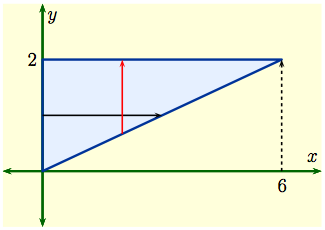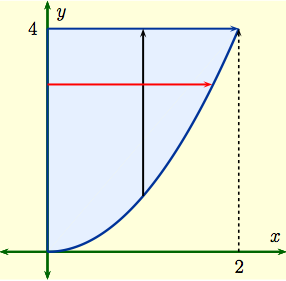Home

#### The Fundamental Theorem of Calculus

Three Different Concepts
The Fundamental Theorem of Calculus (Part 2)
The Fundamental Theorem of Calculus (Part 1)
More FTC 1

#### The Indefinite Integral and the Net Change

Indefinite Integrals and Anti-derivatives
A Table of Common Anti-derivatives
The Net Change Theorem
The NCT and Public Policy

#### Substitution

Substitution for Indefinite Integrals
Examples to Try
Revised Table of Integrals
Substitution for Definite Integrals
Examples

#### Area Between Curves

Computation Using Integration
To Compute a Bulk Quantity
The Area Between Two Curves
Horizontal Slicing
Summary

#### Volumes

Slicing and Dicing Solids
Solids of Revolution 1: Disks
Solids of Revolution 2: Washers
More Practice

#### Integration by Parts

Integration by Parts
Examples
Integration by Parts with a definite integral
Going in Circles

#### Integrals of Trig Functions

Antiderivatives of Basic Trigonometric Functions
Product of Sines and Cosines (mixed even and odd powers or only odd powers)
Product of Sines and Cosines (only even powers)
Product of Secants and Tangents
Other Cases

#### Trig Substitutions

How Trig Substitution Works
Summary of trig substitution options
Examples
Completing the Square

#### Partial Fractions

Introduction
Linear Factors
Improper Rational Functions and Long Division
Summary

#### Strategies of Integration

Substitution
Integration by Parts
Trig Integrals
Trig Substitutions
Partial Fractions

#### Improper Integrals

Type 1 - Improper Integrals with Infinite Intervals of Integration
Type 2 - Improper Integrals with Discontinuous Integrands
Comparison Tests for Convergence

#### Differential Equations

Introduction
Separable Equations
Mixing and Dilution

#### Models of Growth

Exponential Growth and Decay
Logistic Growth

#### Infinite Sequences

Examples of Infinite Sequences
Limit Laws for Sequences
Theorems for and Examples of Computing Limits of Sequences
Monotonic Covergence

#### Infinite Series

Introduction
Geometric Series
Limit Laws for Series
Test for Divergence and Other Theorems
Telescoping Sums and the FTC

#### Integral Test

The Integral Test
Estimates of Value of the Series

#### Comparison Tests

The Basic Comparison Test
The Limit Comparison Test

#### Convergence of Series with Negative Terms

Introduction, Alternating Series,and the AS Test
Absolute Convergence
Rearrangements

The Ratio Test
The Root Test
Examples

#### Strategies for testing Series

Strategy to Test Series and a Review of Tests
Examples, Part 1
Examples, Part 2

#### Power Series

Finding the Interval of Convergence
Power Series Centered at $x=a$

#### Representing Functions as Power Series

Functions as Power Series
Derivatives and Integrals of Power Series
Applications and Examples

#### Taylor and Maclaurin Series

The Formula for Taylor Series
Taylor Series for Common Functions
Adding, Multiplying, and Dividing Power Series
Miscellaneous Useful Facts

#### Applications of Taylor Polynomials

Taylor Polynomials
When Functions Are Equal to Their Taylor Series
When a Function Does Not Equal Its Taylor Series
Other Uses of Taylor Polynomials

#### Partial Derivatives

Visualizing Functions in 3 Dimensions
Definitions and Examples
An Example from DNA
Geometry of Partial Derivatives
Higher Order Derivatives
Differentials and Taylor Expansions

#### Multiple Integrals

Background
What is a Double Integral?
Volumes as Double Integrals

#### Iterated Integrals over Rectangles

How To Compute Iterated Integrals
Examples of Iterated Integrals
Cavalieri's Principle
Fubini's Theorem
Summary and an Important Example

#### Double Integrals over General Regions

Type I and Type II regions
Examples 1-4
Examples 5-7
Order of Integration

### Order of Integration

Some regions can be viewed as both Type I or Type II.  In that case we can set up an iterated integral in two ways.  Depending on the integrand, one can be a lot easier than the other.

Sometimes you're given an impossible-looking iterated integral, and you can solve it by changing the order of integration.  Here are the steps to change the order of integration.

 Begin with an iterated integral that is a double integral over some region $D$. If you are not given $D$, then determine $D$ from the limits of integration. Express $D$ as the other type of region (Type II if it was originally set up as Type I, and Type I if it was originally set up as Type II). Rewrite the integral over $D$ as an interated integral in the other way.  (If had originally been $dx \,dy$, it should now be $dy \,dx$, and vice-versa.) Evaluate the new iterated integral.

Some examples are worked in detail in this video.

 Example 1: Evaluate the integral $$I \ = \iint_D\, x\,\sqrt{1+y^3}\, dA$$ when $D$ is the triangular region shown here, enclosed by the $y$-axis and the lines $$y \ = \ \frac{1}{3}x\,, \qquad y \ = \ 2\,.$$Bad Solution 1:  Fix $x$ and integrate with respect $y$ along the vertical red line. Then $\displaystyle I \ = \ \int_0^6 \left(\int_{x/3}^2\, x\,\sqrt{1+y^3}\, dy\right)\,dx\,.$  The trouble is that the inner integral involves requires evaluating the integral $\displaystyle\int_{x/3}^2\, \sqrt{1+y^3}\, dy\,.$  Nothing you've learned so far in calculus will work here, so we change the order of integration to see if that helps.

Good Solution 1:  Fix $y$ and integrate with respect to $x$ along the black line.  Then $\displaystyle I \ = \ \int_0^2 \left(\int_{0}^{3y}\, x\,\sqrt{1+y^3}\, dx\right)dy\,.$  The inner integral has $\sqrt{1+y^3}$ as a constant, and involves evaluating the integral $$\displaystyle\int_{0}^{3y}\, x\sqrt{1+y^3}\, dx\ = \ \Bigl[\,\frac{1}{2}x^2\sqrt{1+y^3}\, \Bigr]_0^{3y} \ = \ \frac{9}{2}y^2\sqrt{1+y^3}\,.$$  In this case, $$I \ = \ \frac{9}{2} \int_0^2\, y^2 \, \sqrt{1+y^3}\,dy\ \overset{\fbox{ \,\,u\,=\,1+y^3,\\ du\,=\,3y^2\,dy\\u(0)=1\\u(2)=9}\\}{=} \frac{3}{2}\int_1^9 u^{1/2}\,du= \frac{3}{2}\frac{2}{3}u^{3/2}\Bigr|_1^{9}=26\,.$$

-------------------------------------------------------------------------------

Reversing the order of integration in a double integral requires creating a graph of the region of integration.  Then it's a matter of algebra and inverse functions.

Example 2:  Reverse the order of integration in the iterated integral $$I \ = \ \int _0^2\left(\int_{x^2}^4\, f(x,\,y)\, dy\right)dx\,.$$

DO:  Graph the region of integration, which we'll call $D$.

 Solution 2:  The region of integration is the set $$D \ = \ \Bigl\{\,(x,\,y) : \, x^2 \le y \le 4\,, \ \ 0 \le x \le 2\,\Bigr\}$$ which we determine from the limits of integration of $I$.  The graph of $D$ is given to the right.  The given iterated integral fixes $x$ and integrates with respect to $y$ along the vertical black line.  To reverse the order of integration we need to fix $y$ and integrate with respect to $x$ along the red line. DO:  Set up the new iterated integral before proceeding.The horizontal red line in graph of $D$ shows the lower limit of integration is $x = 0$.  The red line ends at the parabola $y = x^2$, which can be written as a function of $y$ as $x = \sqrt{y}$; this tells us the upper limit of integration $x =\sqrt{y}$.  We also note that $y$ goes from $y=0$ to $y=4$.  Thus $D$ can also be written as $$D \ = \ \Bigl\{\,(x,\,y) : \, 0 \le x \le \sqrt{\,y }, \ \ 0 \le y \le 4\,\Bigr\}\,.$$ Rewriting $I$ after changing the order of integration, we get $$I \ = \ \int _0^4\left(\int_0^{\sqrt{\,y}}\, f(x,\,y)\, dx\right)\,dy\,.$$

 Warning: The tricky part of swapping the order of integration is rewriting the limits of integration. This involves studying the region of integration. $$\int_a^b \int_{y=g(x)}^{h(x)}\, f(x,y) \,dy\, dx$$ does NOT become $$\int_{g(x)}^{h(x)} \,\int_a^b f(x,y) \,dx\, dy \hbox{ !!}$$